Hypothesis Testing with Two Samples

# 51 Test for Differences in Means: Assuming Equal Population Variances

<!– Insert module text here –> Typically we can never expect to know any of the population parameters, mean, proportion, or standard deviation. When testing hypotheses concerning differences in means we are faced with the difficulty of two unknown variances that play a critical role in the test statistic. We have been substituting the sample variances just as we did when testing hypotheses for a single mean. And as we did before, we used a Student’s t to compensate for this lack of information on the population variance. There may be situations, however, when we do not know the population variances, but we can assume that the two populations have the same variance. If this is true then the pooled sample variance will be smaller than the individual sample variances. This will give more precise estimates and reduce the probability of discarding a good null. The null and alternative hypotheses remain the same, but the test statistic changes to: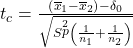where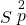is the pooled variance given by the formula: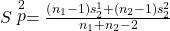A drug trial is attempted using a real drug and a pill made of just sugar. 18 people are given the real drug in hopes of increasing the production of endorphins. The increase in endorphins is found to be on average 8 micrograms per person, and the sample standard deviation is 5.4 micrograms. 11 people are given the sugar pill, and their average endorphin increase is 4 micrograms with a standard deviation of 2.4. From previous research on endorphins it is determined that it can be assumed that the variances within the two samples can be assumed to be the same. Test at 5% to see if the population mean for the real drug had a significantly greater impact on the endorphins than the population mean with the sugar pill.

First we begin by designating one of the two groups Group 1 and the other Group 2. This will be needed to keep track of the null and alternative hypotheses. Let’s set Group 1 as those who received the actual new medicine being tested and therefore Group 2 is those who received the sugar pill. We can now set up the null and alternative hypothesis as:

H0: µ1 ≤ µ2
H1: µ1 > µ2

This is set up as a one-tailed test with the claim in the alternative hypothesis that the medicine will produce more endorphins than the sugar pill. We now calculate the test statistic which requires us to calculate the pooled variance,using the formula above.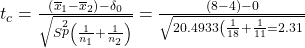tα, allows us to compare the test statistic and the critical value.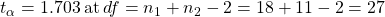The test statistic is clearly in the tail, 2.31 is larger than the critical value of 1.703, and therefore we cannot maintain the null hypothesis. Thus, we conclude that there is significant evidence at the 95% level of confidence that the new medicine produces the effect desired.

### Chapter Review

In situations when we do not know the population variances but assume the variances are the same, the pooled sample variance will be smaller than the individual sample variances.

This will give more precise estimates and reduce the probability of discarding a good null.

### Formula Reviewwhereis the pooled variance given by the formula: